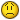# Range issue...

G

#### Guest

Today you've answered several questions in regards to finding the end of a
column and assigning it to a variable. I've attempted to use the information
in these posts on the board and have been unable to make it work in my
instance.

I am doing some regression with the Analysis Toolpack and am trying to
program a macro that will automatically look at how long a column is an
assign that cell to a range.

For instance, in the code below, I'd like to replace the two statements
"ActiveSheet.Range("G6:G135")" as well as the one with the H6:H135 range with
two new statements that generally states "ActiveSheet.Range("G6last
occupied cell in each column")".

..
..
..
Application.Run "ATPVBAEN.XLA!Regress", ActiveSheet.Range("G6:G135"),
ActiveSheet.Range("H6:H135"), False, True, , _
"Weibull-LineToTheData", False, False, False, True, , False
..
..
..

James

G

#### Guest

Hi,

Application.Run "ATPVBAEN.XLA!Regress", ActiveSheet.Range("G6:G" &
Cells(Rows.Count, "G").End(xlUp).Row), _
ActiveSheet.Range("H6:H" & Cells(Rows.Count, "H").End(xlUp).Row), False,
True, , _
"Weibull-LineToTheData", False, False, False, True, , False

HTH

B

#### Bob Phillips

With ActiveSheet
Set rngG = .Range("G6:G" & .Cells(Rows.Count,"G").End(xlUp).Row)
Set rngH = .Range("H6:H" & .Cells(Rows.Count,"H").End(xlUp).Row)
Application.Run "ATPVBAEN.XLA!Regress", rngG,
.rngH,False, True, , _
"Weibull-LineToTheData", False, False, False, True, , False
End With

--

HTH

RP
(remove nothere from the email address if mailing direct)

G

#### Guest

Thanks! Worked like a charm!

Toppers said:
Hi,

Application.Run "ATPVBAEN.XLA!Regress", ActiveSheet.Range("G6:G" &
Cells(Rows.Count, "G").End(xlUp).Row), _
ActiveSheet.Range("H6:H" & Cells(Rows.Count, "H").End(xlUp).Row), False,
True, , _
"Weibull-LineToTheData", False, False, False, True, , False

HTH

G

#### Guest

Thanks for the input!

Bob Phillips said:
With ActiveSheet
Set rngG = .Range("G6:G" & .Cells(Rows.Count,"G").End(xlUp).Row)
Set rngH = .Range("H6:H" & .Cells(Rows.Count,"H").End(xlUp).Row)
Application.Run "ATPVBAEN.XLA!Regress", rngG,
.rngH,False, True, , _
"Weibull-LineToTheData", False, False, False, True, , False
End With

--

HTH

RP
(remove nothere from the email address if mailing direct)